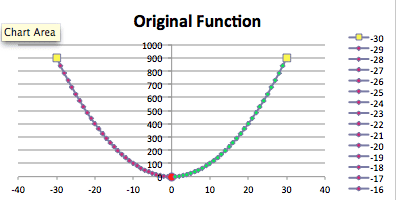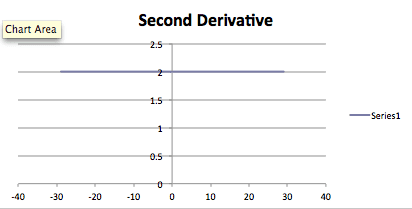# GraphingCalculator

VBScript Graphing Calculator

This is a graphing application that performs Calculus operations based on input functions and generates the graphs of those functions. It was programmed using the VBScript language. The most difficult aspects of this project included the maxima and minima of derivative tests and the colors associated with each aspect of the graphs.

##### Test examples

Below is the generated graph of the input function y=x^2 using this application:The corresponding first derivative:And the corresponding second derivative:This graphing application is extremely useful when a physical graphing calculator and/or internet is unavailable. Enjoy!